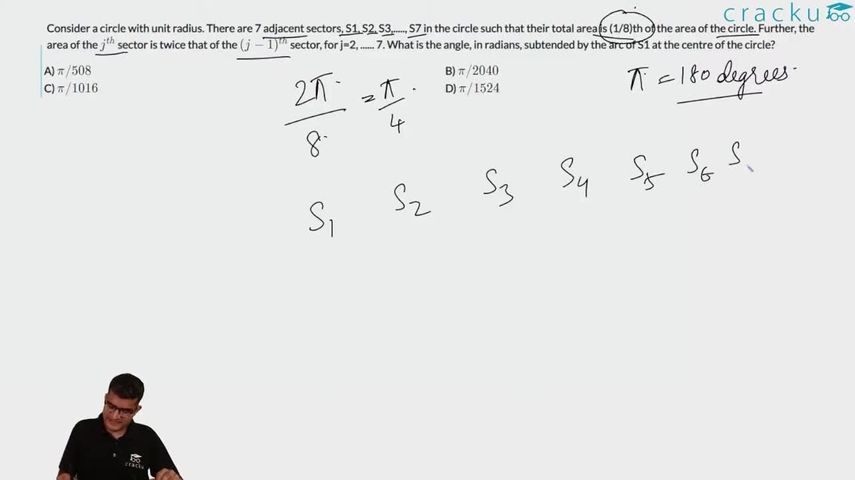Question 147

# Consider a circle with unit radius. There are 7 adjacent sectors, S1, S2, S3,....., S7 in the circle such that their total area is (1/8)th of the area of the circle. Further, the area of the $$j^{th}$$ sector is twice that of the $$(j-1)^{th}$$ sector, for j=2, ...... 7. What is the angle, in radians, subtended by the arc of S1 at the centre of the circle?

Solution

Now area of 1st sector = $$\pi * r^2 * \frac{x}{360}$$ where x - angle subtended at center

Now the next sector will have 2x as the angle, and similarly angles will be in GP with ratio = 2.

Sum of areas of all 7 sectors = $$\frac{127*x* \pi * r^2}{360}$$ which is equal to $$\frac{\pi * r^2}{8}$$

We get x = $$\frac{360}{8*127}$$

Now if converted in radians we get  x = $$\pi/508$$.

### View Video Solution• All Quant CAT Formulas and shortcuts PDF
• 30+ CAT previous papers with solutions PDF

##### Prince Verma

3 years, 7 months ago

Why it is not pi upon 1016?

##### Gunjan Chaudhary

1 month, 4 weeks ago